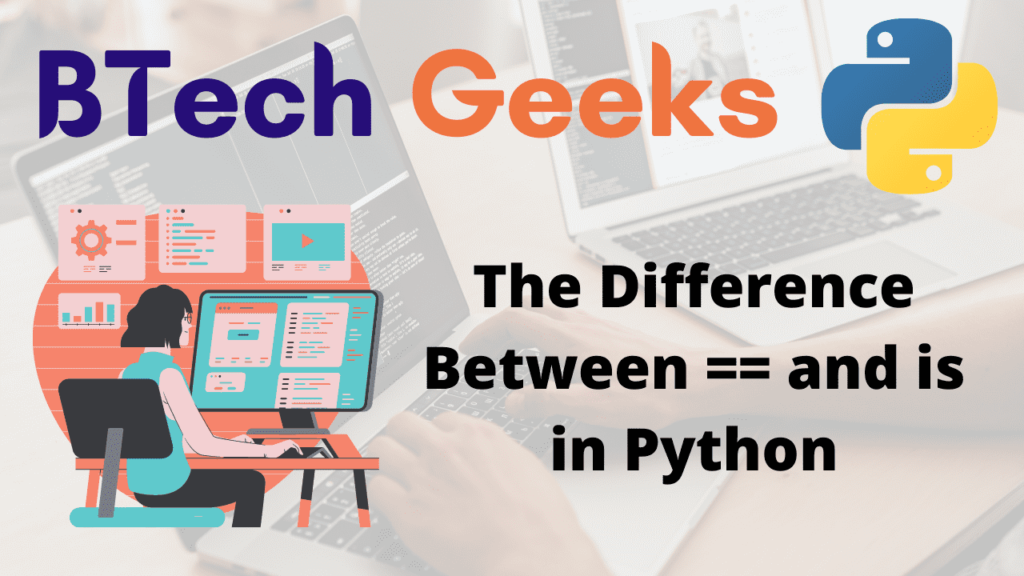# The Difference Between == and is in Python

In Python, the operators == and is do very similar functions, yet they are extremely different and deal with a very important concept: how Python saves its variables in memory.

There is a small distinction between the Python identity operator (is) and the equality operator (==). When you use the Python is operator to compare numbers, your code may work great until it suddenly does not. You may have heard that the Python is operator is faster than the == operator, or you may believe that it appears more Pythonic. However, it is critical to remember that these operators do not behave in the same way.

The Python is operator checks if two variables link to the same object in memory, whereas the == operator compares the value or equality of two objects. Except when comparing to None, which means you should utilise the equality operators == and!= in the vast majority of situations.

## Understanding the difference Between == and ‘is’ in Python

Explore more instances related to python concepts from Python Programming Examples Guide and get promoted from beginner to professional programmer level in Python Programming Language.

### Difference #1: Implementation

== compares the values of the two operands and returns True if they are the same, False otherwise.
is compares the object IDs of the two operands and returns True if they match.

But what exactly is an object ID? In memory, each object is assigned an ID, and two variables can point to the same item, giving them the same object ID.

listone = ["this", "is", "BTechGeeks"]
listtwo = ["this", "is", "BTechGeeks"]
print(listone == listtwo)
print(listone is listtwo)
# assigning list two with list one
listtwo = listone
print("After assigning list two with list one:")
# using is
print(listone is listtwo)


Output:

True
False
After assigning list two with list one:
True

In the preceding example, there are two lists in memory at first, but they contain the identical values.

== compares values and returns True if the operation is successful.
However, it tests to see if they point to the same object, and because these lists do not link to the same object, it returns False.
After that, we set listwo equal to listone, which causes listwo to point to where listone is pointing, and it returns True.
All of this is extremely different for integers, and in order to grasp this, we must first understand how objects are stored in memory.

### Difference #2:Memory Allocation

Let us take a number and initialize it with 6 as below

number=6

We know the number has the value 6, but how does it become stored in memory? In Python, each object has four memory parts:

Size — For each object, four bytes are reserved to store the object’s size.
Reference Count – For each object, 8 bytes are reserved to store the number of variables pointing to this object. And all of these objects will have this object’s object ID.
Object Type — For each object, 8 bytes are reserved to retain information indicating the type of object.
Object Value — An additional 8 bytes are reserved for each item and contain the object’s actual value.

Object value and reference count are the two most significant items in the preceding list for this topic.

So, for number = 6, there is an object in memory with object value 6 and reference count 1, indicating that the object has a value of 6 and one variable, in this case number, is pointing towards it.

Let us now declare another variable as follows:

number = 6
number2 = number
print(number == number2)
print(number is number2)


Output:

True
True

Now, something very fascinating will happen in memory: no new objects will be generated, and number 2 will also point to where number is pointing, and the object’s reference count will increase to 2.

As a result, number and number 2 will have the same object ID, like the above output.

But what if we do as given below?

number = 6
number2 = 6
print(number == number2)
print(number is number2)


Output:

True
True

Related Programs: